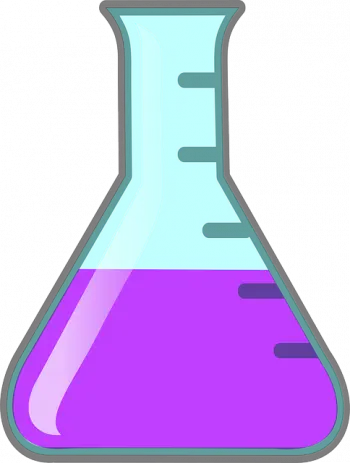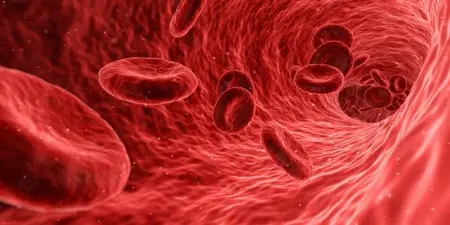# Henry's LawHenry's law is a fundamental principle in chemistry and physics that establishes an important relationship between the concentration of a gas in a solution and its partial pressure in space above the solution.

Named after the British chemist William Henry, who first proposed it in the 19th century, this law has been a crucial tool for understanding and manipulating the behavior of gases dissolved in liquids.

## Definition of Henry's LawHenry's law describes how the concentration of a gas dissolved in a liquid is directly proportional to the partial pressure of the gas in the gas phase above the solution. In other words, at a constant temperature, the concentration of a gas in a solution increases linearly with the pressure of the gas.

The definition of this law is valid only under ideal conditions, that is, when there are no significant interactions between the gas molecules and the solvent molecules, and when the temperature remains constant. Under real conditions, deviations from Henry's Law can occur due to molecular interactions and changes in temperature.

## Formula

This relationship is expressed by the equation:

C = k * P

Where:

• C is the concentration of the gas in the solution which is usually expressed in molarity (moles per liter), so the common unit is molar (M)

• P is the partial pressure of the gas in the gas phase above the solution expressed in atmospheres (atm)

• k is Henry's constant, which depends on the nature of the gas, the solvent and the temperature. Henry's constant will have units of M/atm.

## Henry's Constant

Henry's constant (k) is a specific constant for each gas and solvent at a given temperature. It represents the ratio between the concentration of a gas dissolved in a liquid and its partial pressure in the gas phase above the solution.

Below is a table with examples of Henry's constants for some common substances in water at 25°C:

 Substance Chemical formula Henry's constant (k) in water at 25°C (M/atm) Oxygen O₂ 1.3×10 −3 Carbon dioxide CO₂ 3.3 x 10 -2 Nitrogen N₂ 6.1×10 −4 Hydrogen H₂ 7.6 x 10 -4 Ammonia NH₃ 5.6×10 −2 Methane CH₄ 1.2×10 −3 Sulfur dioxide SO₂ 5.6×10 −2

## Examples

Here are some examples of Henry's Law in action:

### Example 1: Oxygen Dissolved in Water

Suppose we have a container of water at 25°C and we are exposing the surface of the water to atmospheric air, which contains oxygen (O₂) at a partial pressure of 0.21 atm (the mole fraction of oxygen in air).

According to Henry's law, the concentration of dissolved oxygen in water will increase directly proportional to the partial pressure of oxygen. If Henry's constant for oxygen in water is 1.3×10 −3  M/atm (as mentioned in the table above), we can calculate the concentration of oxygen in water:

O₂ concentration = k * P

O₂ concentration = (1.3×10−3  M/atm) * (0.21 atm) = 2.73×10−4  M

Therefore, the concentration of dissolved oxygen in water will be approximately 2.73×10−4 moles per liter.

### Example 2: Carbon Dioxide in a Carbonated DrinkIn a carbonated beverage like soda, carbon dioxide (CO₂) dissolves in the liquid under high pressure during the carbonation process. As the can or bottle is opened, the pressure on the drink decreases and the CO₂ begins to escape as bubbles.

The amount of CO₂ dissolved in the drink follows Henry's law. The greater the pressure on the beverage (such as when it is hermetically sealed), the greater the concentration of dissolved CO₂. When the pressure is released (by opening the can), the CO₂ is released as bubbles.

### Example 3: Solubility of Nitrogen in Water

Nitrogen (N₂) is another gas that shows Henry's law in action. At low temperatures and high pressures, nitrogen can dissolve in water in significant quantities.

For example, in the manufacture of soft drinks, high-pressure liquid nitrogen is used to impregnate liquids with dissolved nitrogen. As the pressure decreases, nitrogen is released, which can create bubbles and interesting visual effects.

## Scientific ApplicationsHenry's law has a wide range of applications in various fields, including chemistry, biology, chemical engineering and geology.

Some of its most notable applications include:

• Analytical chemistry – In analytical chemistry, it is used to determine the concentration of a specific gas in a liquid sample.
• Biology and physiology : in this field it is applied to the law of ideal gases and respiration. For example, in human blood, the concentration of dissolved oxygen and carbon dioxide follows Henry's law, which is essential for the transport of oxygen from the lungs to the tissues and the elimination of carbon dioxide.
• Chemical engineering : In the chemical and process industry, it is used to design and operate gas absorption and desorption systems.
• Geology : In geology it is applied to the study of the solubility of gases in geological liquids, such as the solubility of gases in groundwater.

## Limitations and Deviations of Henry's Law

Despite its usefulness, Henry's law has important limitations.

One of the main limitations is that it is not valid at all temperatures and pressures. At high pressures and low temperatures, the interactions between the gas molecules and the solvent become significant, which can result in significant deviations from the law.

Additionally, some gases may experience limited solubility in certain solvents due to the formation of chemical reactions or the occurrence of phase changes.

Author:

Published: September 27, 2023
Last review: November 30, 2023